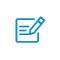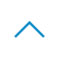•• 注册 忘记密码?

# Pytorch中的四种经典Loss源码解析

MarginRankingLoss
RankingLoss系列是来计算输入样本的距离，而不像MSELoss这种直接进行回归。其主要思想就是分为 Margin 和 Ranking。

MarginRankingLoss公式
Margin 这个词是页边空白的意思，平常我们打印的时候，文本内容外面的空白就叫 Margin。

Ranking 则是排序，当target=1，则说明x1排名需要大于x2；当target=2，则说明x2排名需要大于x1。

Pytorch的MarginRankingLoss代码

def np_margin_ranking_loss(input1, input2, target, margin, reduction):
output = np.maximum(0, -target*(input1 - input2) + margin)
if reduction == "mean":
return np.mean(output)
elif reduction == "sum":
return np.sum(output)
else:
return output

TripletMarginLoss
TripletLoss最早是在 FaceNet 提出的，它是用于衡量不同人脸特征之间的距离，进而实现人脸识别和聚类

TripletLoss

## 伪代码
if swap:
D(a, n) = min(D(a,n), D(p, n))

TripletMarginLoss源码
at::pairwise_distance是距离计算函数，首先计算出了anchor与正类和负类的距离。 然后根据参数swap，来确定是否考虑正类和负类之间的距离。最后output就是按照公式进行计算，下面是numpy的对应代码
def np_triplet_margin_loss(anchor, postive, negative, margin, swap, reduction="mean", p=2, eps=1e-6):
def _np_distance(input1, input2, p, eps):
# Compute the distance (p-norm)
np_pnorm = np.power(np.abs((input1 - input2 + eps)), p)
np_pnorm = np.power(np.sum(np_pnorm, axis=-1), 1.0 / p)
return np_pnorm

dist_pos = _np_distance(anchor, postive, p, eps)
dist_neg = _np_distance(anchor, negative, p, eps)

if swap:
dist_swap = _np_distance(postive, negative, p, eps)
dist_neg = np.minimum(dist_neg, dist_swap)
output = np.maximum(margin + dist_pos - dist_neg, 0)

if reduction == "mean":
return np.mean(output)
elif reduction == "sum":
return np.sum(output)
else:
return output

KLDivLoss源码

_kl_div_log_target 的实现比较简单，就是按照公式进行计算

def np_kldivloss(input, target, log_target, reduction="mean"):
if log_target:
output = np.exp(target)*(target - input)
else:
output_pos = target*(np.log(target) - input)
zeros = np.zeros_like(input)
output = np.where(target>0, output_pos, zeros)
if reduction == "mean":
return np.mean(output)
elif reduction == "sum":
return np.sum(output)
else:
return output

BCEWithLogitsLoss

BCEWithLogitsLoss参数
weight 表示最后loss缩放权重
reduction 表示 最后是做mean, sum, none 操作
pos_weight 表示针对正样本的权重，即positive weight

BCEWithLogitsLoss
BCEWithLogitsLoss 相当于 sigmoid + BCELoss，但实际上 Pytorch为了更好的数值稳定性，并不是这么做的，下面我们看看对应的源代码

Pytorch的BCEWithLogitsLoss源码

def np_bce_with_logits_loss(np_input, np_target, np_weight, np_pos_weight, reduction="mean"):
max_val = np.maximum(-np_input, 0)

if np_pos_weight.any():
log_weight = ((np_pos_weight - 1) * np_target) + 1
loss = (1 - np_target) * np_input
loss_1 = np.log(np.exp(-max_val) + np.exp(-np_input - max_val)) + max_val
loss += log_weight * loss_1
else:
loss = (1 - np_target) * np_input
loss += max_val
loss += np.log(np.exp(-max_val) + np.exp(-np_input - max_val))

output = loss * np_weight

if reduction == "mean":
return np.mean(output)
elif reduction == "sum":
return np.sum(output)
else:
return output

triplet-loss(https://omoindrot.github.io/triplet-loss)
FaceNet(https://arxiv.org/pdf/1503.03832.pdf)
TripletMarginLoss(http://www.bmva.org/bmvc/2016/papers/paper119/paper119.pdf)
RankingLoss(https://gombru.github.io/2019/04/03/ranking_loss/)Copyright©2005-2020 Sykv.com 可思数据 版权所有    京ICP备14056871号-1扫码入群返回顶部